« Exercise 4.9.3 Exercise 4.9.5 » Exercise 4.9.4 Given: Loads and concrete class are the same as in the previous exercise. Question : Perform static analysis to determine deflections for the previous exercise slabs, considering the first two as two-way instead of one-way. See the following figure.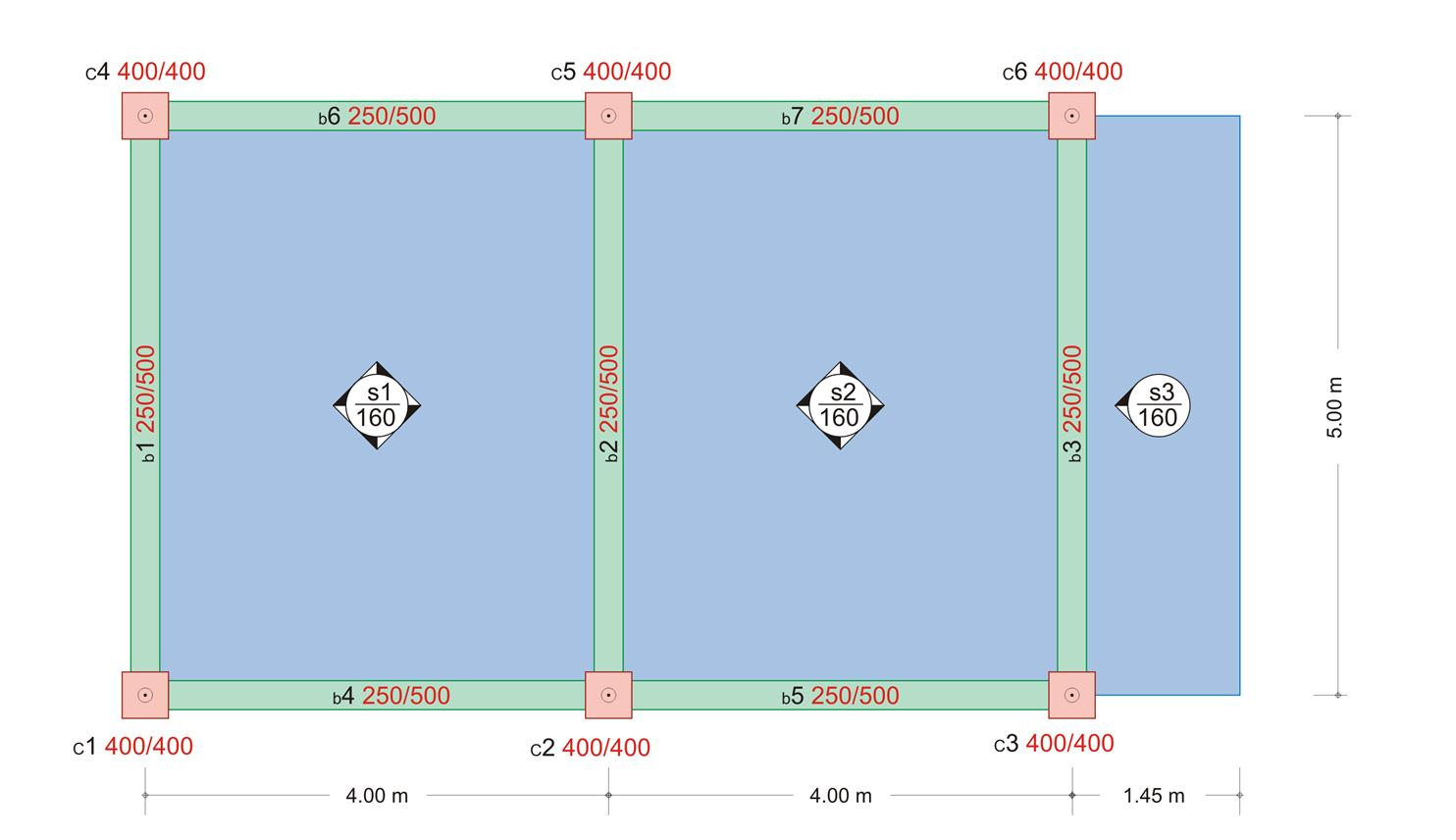Figure 4.9.4-1: Project <Β_494> Figure 4.9.4-1: Project <Β_494> Solution: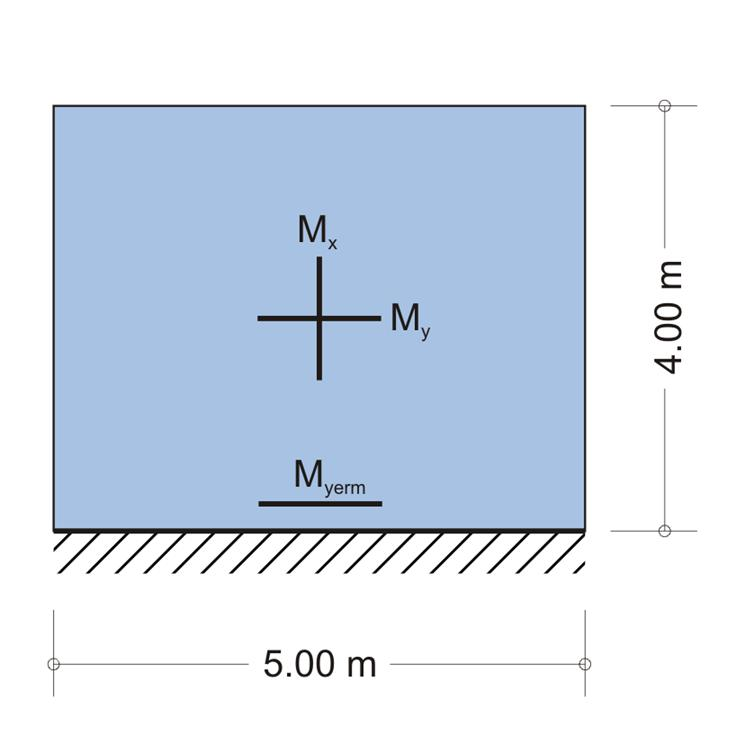Figure 4.9.4-2 Figure 4.9.4-2 Due to the unknown influence of cantilever slab s3 on the adjacent one, for safety reasons, slabs s2 and s1 are considered fixed at one end. s1,s2: consider the slabs rotated by 90º, thus from table b5.2 for ε=ly/lx=4.0/5.0=0.80 akx=0.141, ky=0.859, vx=0.817, vy=0.742, am=0.0180, ρxr=ρyr=0.293, ρyerm=0.507, υxr=0.146, υyr=0.207, υyerm=0.359 a px=kx·p=0.141x9.75=1.37 kN/m, py=ky·p=0.859x9.75=8.38 kN/m Mx=νx·px · lx2/8=0.817x1.37x5.02/8=3.50 kNm My=νy·py · ly2/14.22=0.742x8.38x4.02/14.22=7.00 kNm Myerm=-py·ly2/8=-8.38x4.02/8=-16.76 kNm fm=0.0180x9.75x103N/m2x5.04m4/(35.20x109N/m2x1.0mx0.163m 3)==761x10-6m=0.76 mm Vxr=Vyr=ρxr·p·lx=0.293x9.75x5.0=14.28 kN Vyerm=ρyerm·p·lx=0.507x9.75x5.0=24.72 kN pxr=υxr·p·lx=0.146x9.75x5.0=7.12 kN/m pyr=υyr·p·lx=0.207x9.75x5.0=10.09 kN/m pyerm=υyerm·p·lx=0.359x9.75x5.0=17.50 kN/m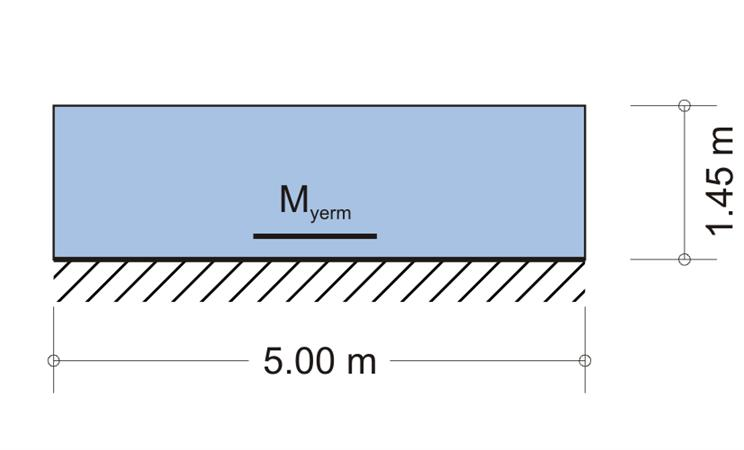Figure 4.9.4-3 Figure 4.9.4-3 Support s1-s2: M1-2=-(16.76+16.76)/2=-16.76 kNm Support s2-s3: Μ2-3=Myerm,3=-p·ly2/2-P·ly=-14.25x1.452/2-1.35x1.45=-16.94 kNm Vyerm,3=p·ly+P=14.25x1.45+1.35=22.01 kN The deflection of the cantilever end is determined by the expression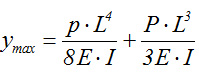, where the first term is due to the uniform load and the second to the concentrated load applied at its end. In case of a slab (i.e. a strip of width b=1.0m), where I=b·h3/12, the maximum deflection (at the end) is: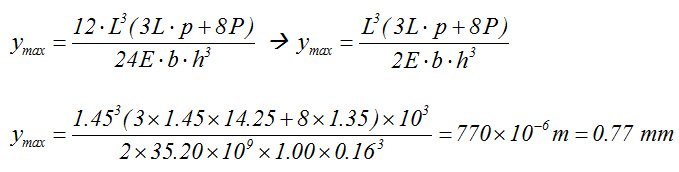Results are transferred to the initial coordinate system producing the following stress resultants: M1-2=-16.76, M2-3=-16.94, Mx,1=7.00, My,1=3.50, Mx,2=7.00, My,2=3.50 [kNm] Vxr,1=14.28, Vxerm,1=24,72, Vyr,1=14.28, Vxerm,2=24.72, Vxr,2=14.28, Vyr,2=14.28, V xerm,3=22.01 [kN] the following equivalent uniform support reactions: pxr,1=10.09, pxerm,1=17.50, pyr,1=7.12, pxerm,2=17.50, pxr,2=10.09, pyr,2=7.12 [kN/m] and loads on beams: b1: p=10.09 kN/m, b4 and b6: p=7.12, b2: p=17.50+17.50=35.00, b5 and b7: p=7.12 b3:p=10.44+22.01=32.45 [kN/m] « Exercise 4.9.3 Exercise 4.9.5 »